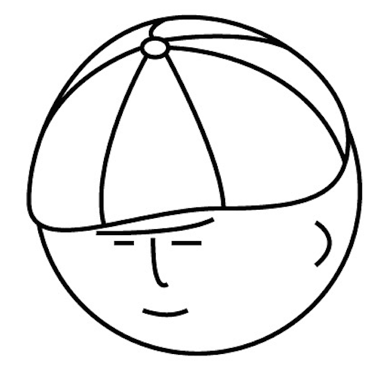マツシタのお勉強メモ

マツシタのお勉強

welcome to my engineering blog
Hacker RankCompress a String

Problem Implement a method to perform basic string compression using the counts of repeated characters. For example, the string aabcccccaaa would become a2b1c5a3. If the string would not become smaller than the original string, your method…

Replace all space in a string with '%20'

Problem Write a method to replace all space in a string with '%2d'. You may assume that the string has sufficient space at the end of the string to hold the additional character, and that you are given the true length of the string. [examp…

If the string is a permutation of another string

Problem Given two strings, write a method to decide if one is a permutation of the other. How to Solve In oder to count of the number that how many times each characters appeared, I use array like HashMap. In case the given strings is ASCI…

Implementation Reverse String Function

How to Implement By swapping the head of the string character and the tail of one, I can reverse a string

Determine if a string has all unique characters

Problem Implement an algorithm to determine if a string has all unique characters. What if you cannot use additional data structures. How to solve Character can be transformed into integer. So, by using array its size is 256(ASCII), we can…

Implementation of "Ring Buffer" in Java

What's "Ring Buffer" Ring Buffer is basically like Queue. The difference between Ring Buffer and normal Queue is that Ring Buffer is circular data structure. So, if tail and head index reach the index of max size, they are reseted zero. Th…